“Onion Ring Bokeh”¶

In the photography community, defects in the out-of-focus highlights of lenses are widely discussed. These result from mid-particular spatial frequency errors on the optical surfaces. Here, we will show an example of using prysm in a relatively extended fashion to model this. We begin, as usual, by importing some classes and other libraries.

:
import numpy as np

from prysm import NollZernike, PSF

from matplotlib import pyplot as plt
%matplotlib inline

We begin by using the NollZernike class to make a pupil with 25 waves fo defocus to give us a relatively uniform disk for the diffraction limited out of focus image.

:
pupil = NollZernike(Z4=25/1.5, samples=384, dia=25)  # 100 waves PV of defocus, 25mm for F/2
pupil._gengrid()  # generate the (normalized coordinate) grid, stored in pupil.rho, pupil.phi

ps = PSF.from_pupil(pupil, efl=50, Q=1.25)
ps.plot2d(axlim=200, power=3)
:
(<Figure size 432x288 with 2 Axes>,
<matplotlib.axes._subplots.AxesSubplot at 0x7f644fd9ceb8>)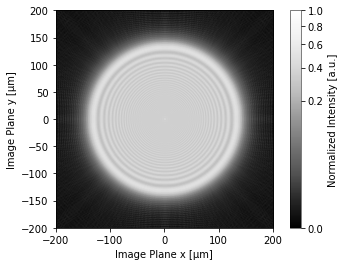The ripples are Fresnel rings and are a consequence interference very similar to the Gibbs phenomenon. They disappear the farther out of focus you go, and can be wiped away by coarser sampling with an image sensor, or significantly polychromatic light.

We used a value of Q below 2 (worse than Nyquist sampled) because the image is so out of focus, it largely lacks high frequency detail to be aliased, so the choice of Q has minimal consequence.

What if the pupil had some ripples in it from structured errors on optical surfaces in the system?

:
pupil2 = pupil.copy()

# 15 cycles per aperture, lambda/25 RMS amplitude
const = 2 * np.pi * 15
phase_mod = np.sin(pupil.rho * const) * (np.sqrt(2) / 25)
pupil2.phase += phase_mod

ps = PSF.from_pupil(pupil2, efl=50, Q=1.25)
ps.plot2d(axlim=200, power=3)
:
(<Figure size 432x288 with 2 Axes>,
<matplotlib.axes._subplots.AxesSubplot at 0x7f644dced2b0>)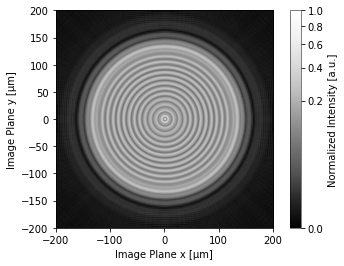The ripples print through into the in-focus image. What if the image was in focus?

:
pupil3 = NollZernike(samples=384, dia=25)  # same parameters as before, but no error
pupil3.phase += phase_mod

pupil3.plot2d(clim=0.25)  # +/- 138 nm
plt.title('Wavefront with ripple error')

ps = PSF.from_pupil(pupil3, efl=50, Q=3) # Q=2 now, we need high resolution
ps.plot2d(axlim=20, power=4)
:
(<Figure size 432x288 with 2 Axes>,
<matplotlib.axes._subplots.AxesSubplot at 0x7f644c3c2710>)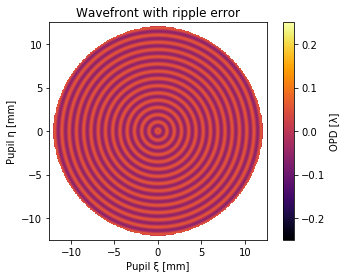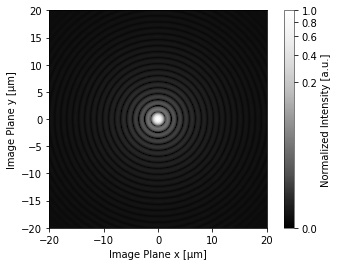This looks just like an Airy disk; the ripples have low RMS amplitude, so they do little damage to the in-focus image.

What if the ripples were more localized, occuring in just one band within the clear aperture?

:
def gauss_r(r, center, sigma=0.1, amplitude=1):
numerator = (r-center) ** 2
denominator = 2 * sigma ** 2
return amplitude * np.exp(-numerator / denominator)

phase_mod2 = phase_mod * gauss_r(pupil.rho, center=0.66, sigma=0.02)
phase_mod_vis = phase_mod2.copy()
plt.imshow(phase_mod_vis, cmap='inferno')

pupil4 = pupil.copy()
pupil4.phase += phase_mod2

ps = PSF.from_pupil(pupil4, efl=50, Q=1.25) # Q=2 now, we need high resolution
ps.plot2d(axlim=200, power=3)
:
(<Figure size 432x288 with 2 Axes>,
<matplotlib.axes._subplots.AxesSubplot at 0x7f644c250518>)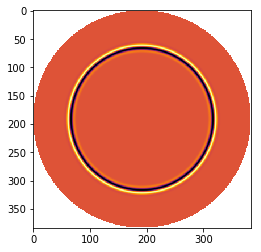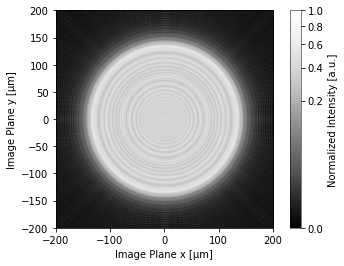Now there is an inner ring in addition to the Fresnel rings. The formation of this is related to the angular spectrum of the aperture. An ensemble of rays from the perturbed annulus of the aperture propagate at a different angle to the rest; the interference begins at the radius they appear at within the clear aperture, crosses through the center at focus where they overlap with the natural interference from the support of the aperture, then dissipate to an infinite distance as the observation plane moves further behind focus.